• 是否可以从matlab中打开Excel中的工作表并编辑公式？这个想法是通过创建第二张表来自动化不确定性分析,其中每个单元格中的不确定性来自前一个单元格的值.基本上,我想将单元格视为变量,并为每个单元格执行SQRT(SUM...

是否可以从matlab中打开Excel中的工作表并编辑公式？这个想法是通过创建第二张表来自动化不确定性分析,其中每个单元格中的不确定性来自前一个单元格的值.基本上,我想将单元格视为变量,并为每个单元格执行SQRT(SUM(Partials(xi)^ 2)). matlab应该没有问题,但它可以编辑表中的公式吗？

目前的过程是从excel复制并粘贴到matlab.这是一个小函数,可以在matlab中对方程组进行不确定性：

function [f_u_total f_u] = uncertAnalysis(f, vars, vars_u)

f_u = [];

f_u_total = [];

for(i=1:length(f))

f(i)

item = uncertAnalysisi(f(i), vars, vars_u);

f_u = [f_u; item(1)];

f_u_total = [f_u_total; item(1)];

end

end

function [f_u_total f_u] = uncertAnalysisi(f, vars, vars_u)

f_u = [];

% take the partials and square them

for i=1:length(vars)

f_u = [f_u; vars(i) (diff(f, vars(i)).*vars_u(i)).^2];

end

f_u_total = (sum(f_u(:,2))).^.5;

end

顺便说一下,方程看起来像这样(为什么我不是手工做)：

=(9*C!S3^2/C!V3^4*C!W3*(C!O3-

C!P3)/C!X3*C!Q3^6*C!F3^4/C!Y3^6/(C!U3^C!Z3)^6*F3^2+1/4*C!S3^2/C!V3^4*C!W3/(C!O3-

C!P3)/C!X3*C!Q3^6*C!F3^6/C!Y3^6/(C!U3^C!Z3)^6*O3^2+1/4*C!S3^2/C!V3^4*C!W3/(C!O3-

C!P3)/C!X3*C!Q3^6*C!F3^6/C!Y3^6/(C!U3^C!Z3)^6*P3^2+9*C!S3^2/C!V3^4*C!W3*(C!O3-

C!P3)/C!X3*C!Q3^4*C!F3^6/C!Y3^6/(C!U3^C!Z3)^6*Q3^2+1/C!V3^4*C!W3*(C!O3-

C!P3)/C!X3*C!Q3^6*C!F3^6/C!Y3^6/(C!U3^C!Z3)^6*S3^2+9*C!S3^2/C!V3^4*C!W3*(C!O3-

C!P3)/C!X3*C!Q3^6*C!F3^6/C!Y3^6/(C!U3^C!Z3)^6*C!Z3^2/C!U3^2*U3^2+4*C!S3^2/C!V3^6*C!W3*(C!O

3-C!P3)/C!X3*C!Q3^6*C!F3^6/C!Y3^6/(C!U3^C!Z3)^6*V3^2+1/4*C!S3^2/C!V3^4/C!W3*(C!O3-

C!P3)/C!X3*C!Q3^6*C!F3^6/C!Y3^6/(C!U3^C!Z3)^6*W3^2+1/4*C!S3^2/C!V3^4*C!W3*(C!O3-

C!P3)/C!X3^3*C!Q3^6*C!F3^6/C!Y3^6/(C!U3^C!Z3)^6*X3^2+9*C!S3^2/C!V3^4*C!W3*(C!O3-

C!P3)/C!X3*C!Q3^6*C!F3^6/C!Y3^8/(C!U3^C!Z3)^6*Y3^2+9*C!S3^2/C!V3^4*C!W3*(C!O3-

C!P3)/C!X3*C!Q3^6*C!F3^6/C!Y3^6/(C!U3^C!Z3)^6*LOG(C!U3)^2*Z3^2)^(1/2)

展开全文• 创建新的 Excel 文件（或如果文件存在则打开它）并按照 (sheetnames) 中列出的方式命名工作表并将工作簿另存为 (filename)。 xlsheets（工作表名，文件名） xlsheets(sheetnames) sheetnames：工作表名称列表...matlab
• xlsread和xlswrite没提供对sheet的操作，从ActiveX来处理新增sheete = actxserver('Excel.Application'); % # open Activex %serverewb = e.Workbooks.Open(name); % # open file (enter full path!)ewb.Worksheets....

新增sheet

e = actxserver('Excel.Application'); % # open Activex %server

ewb = e.Workbooks.Open(name); % # open file (enter full path!)

ewb.Save % # save to the same file

ewb.Close(false)

e.Quit1

2

3

4

5

6

打开name文件，注意要完整的路径。在原先基础上新增一个sheet，一个新的exl是3个sheet。

对sheet重命名

e = actxserver('Excel.Application'); % # open Activex server

ewb = e.Workbooks.Open(name); % # open file (enter full path!)

ewb.Worksheets.Item(num).Name = mn; % # rename num sheet

ewb.Save % # save to the same file

ewb.Close(false)

e.Quit1

2

3

4

5

6

对第num个sheet进行重命名

贴一部分代码，实现的是，新建tg为文件名的xls，文件的sheet分别为method。xlswrite 写cell 文件方便。

function Untitled2()

set = {'ECSSD'};

method = {'VA', 'SO'};

methodv = {'MSE', 'PR'};

tg = {'J:\ECSSD.xls','J:\MSRA1000.xls'};

setup(tg{1}, method);setup(tg{2}, method);

end

function str = double2mystr(a)

str = sprintf('%.4f%%',a*100);

end

% 新建一个文件，以method为sheet

function setup(name, method)

% 初始化 name 文件

xlswrite(name, 1);

for i1 = 1 : length(method)

mn = method{i1};

% 使用 ActiveX 来增加sheet

sheetn(name, i1, mn);

end

end

function sheetn(name, num, mn)

e = actxserver('Excel.Application'); % # open Activex server

ewb = e.Workbooks.Open(name); % # open file (enter full path!)

if num > 3

end

ewb.Worksheets.Item(num).Name = mn; % # rename 1st sheet

ewb.Save % # save to the same file

ewb.Close(false)

e.Quit

end1

2

3

4

5

6

7

8

9

10

11

12

13

14

15

16

17

18

19

20

21

22

23

24

25

26

27

28

29

30

31

32

33

34

35

36

展开全文• MATLAB生成excel表格

万次阅读 多人点赞 2018-11-13 18:26:00
利用excel对数据进行复杂处理有一定局限性，于是选择导入MATLAB进行处理。此贴针对MATLAB将数据导出至excel，列举一些简单的例子，帮助大家学习。

by HPC_ZY

核心函数

xlswrite(filename, data);
其中，data可以是二维数组（mat）或二维元胞（cell）
详细参数可在命令行窗口中输入以下代码查看

help xlswrite

实例

常规

1. 普通数组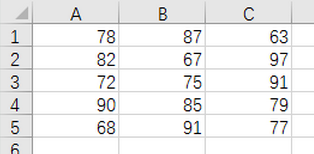当表格只有数字时，可以直接存储矩阵
nummat=round(rand(5,3)*40+60);
xlswrite('mat.xls',nummat);

1. 文字矩阵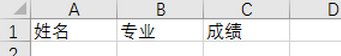strmat={'姓名','专业','成绩'};
xlswrite('str.xls',strmat);

1. 混合内容
创建以下表格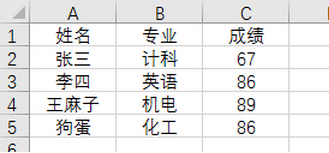% 初始化结果（使用cell结构，与输出同尺寸，每个元素对应Excel一个格子）
data1 = cell(5,3);
% 建立表头
title = {'姓名','专业','成绩'};
% 建立数据
name = {'张三';'李四';'王麻子';'狗蛋'};
major = {'计科';'英语';'机电';'化工'};
score = round(rand(4,1)*40+60);
% 格式调整(普通数组通过以下函数转为同尺寸cell格式)
score = num2cell(score);
% 整合
data1(1,:)=title;
data1(2:end,1)=name;
data1(2:end,2)=major;
data1(2:end,3)=score;
xlswrite('data1.xls',data1);

特殊

1. 输出多个sheet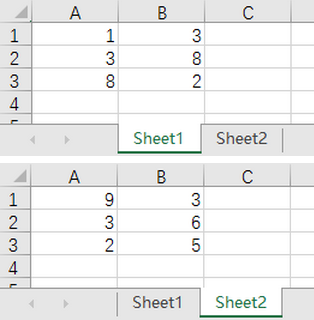num1=round(10*rand(3,2));
num2=round(10*rand(3,2));
xlswrite('file1.xls',num1,1)
xlswrite('file1.xls',num2,2)

1. 在指定位置生成表格数据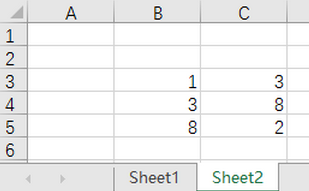num=round(10*rand(3,2));
sheet = 2;
pos='B3';
xlswrite('file2.xls',num,sheet,pos)

其他

1. 更新了一篇《多个excel表合成一个
展开全文MATLAB excel
• matlab生成excel文档源码

千次阅读 2019-02-16 17:09:45
1. 创建一个本地的Excel服务端,其实就是打开Excel try Excel = actxGetRunningServer('Excel.Application'); catch Excel = actxserver('Excel.Application'); end 表达意思如下 try 如果Excel 服务器已经...

目录

1. 创建一个本地的Excel服务端,其实就是打开Excel

2. 让打开的Excel可见

3. 创建一个工作簿

4. 创建一个表单

5.页面设置

6. 设置行高列宽

7.单元格子合并

8.设置单元格的边框

9.设置单元格对齐方式

10. 写入单元格内容（Value）

11.设置字号样式

12. 保存WorkBook和退出Excel服务端

1. 创建一个本地的Excel服务端,其实就是打开Excel

try
Excel = actxGetRunningServer('Excel.Application');
catch
Excel = actxserver('Excel.Application');
end

表达意思如下

try

如果Excel 服务器已经打开，返回其句柄

catch

如果Excel服务器没有打开，则创建一个Excel服务器，并返回句柄

end

2. 让打开的Excel可见

默认情况下启动服务器是不可见的，Excel.Visible1时可见，为0时候不可见。

Excel.Visible = 1;%设置为看见或者set(Excel, 'Visible', 1);
Excel.Visible = 0;%设置为看不见或者set(Excel, 'Visible', 0);

经过1-2两步后效果如下图1图1

3. 创建一个工作簿

也就是创建一个空白的Excel文件效果如下图2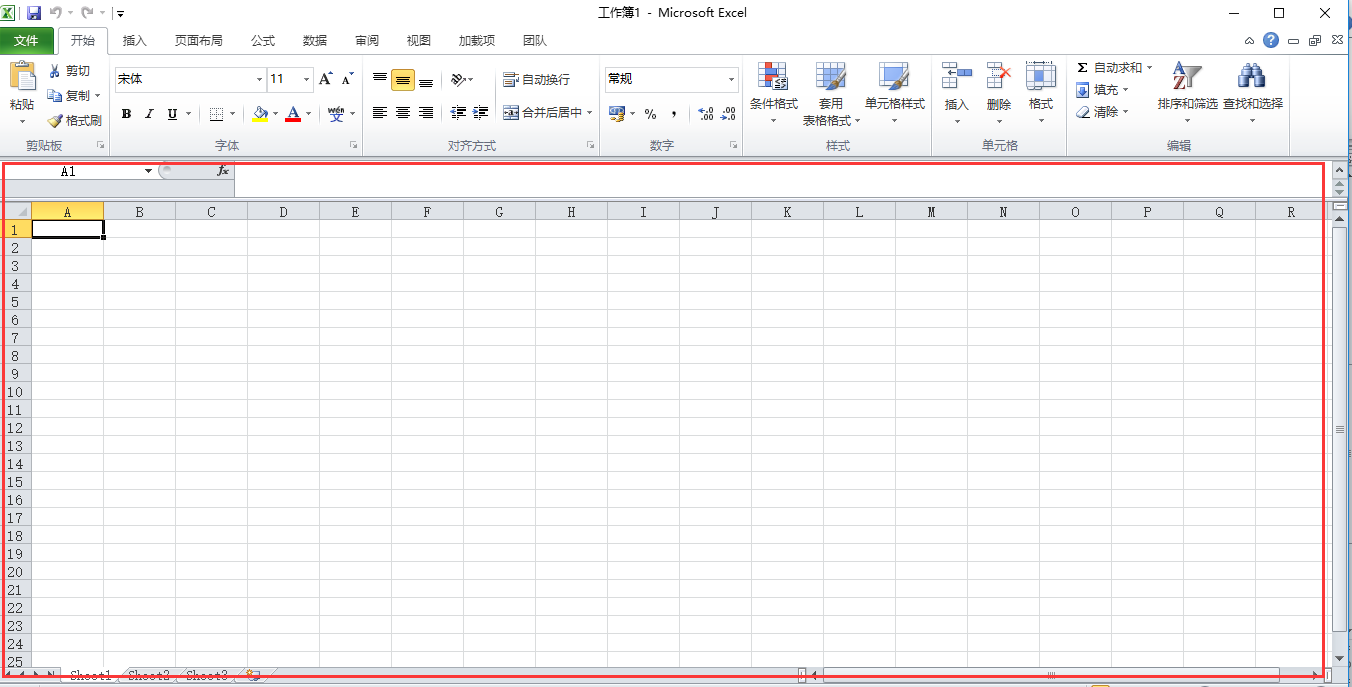图2

4. 创建一个表单

Sheet1 = WorkBook.Sheets.Item(1);%表单句柄

表单创建好后需要激活，还需要给表单起个名

Sheet1.Activate;%激活
Sheet1.Name = '我是第一个表单';%起名字

效果图如下图3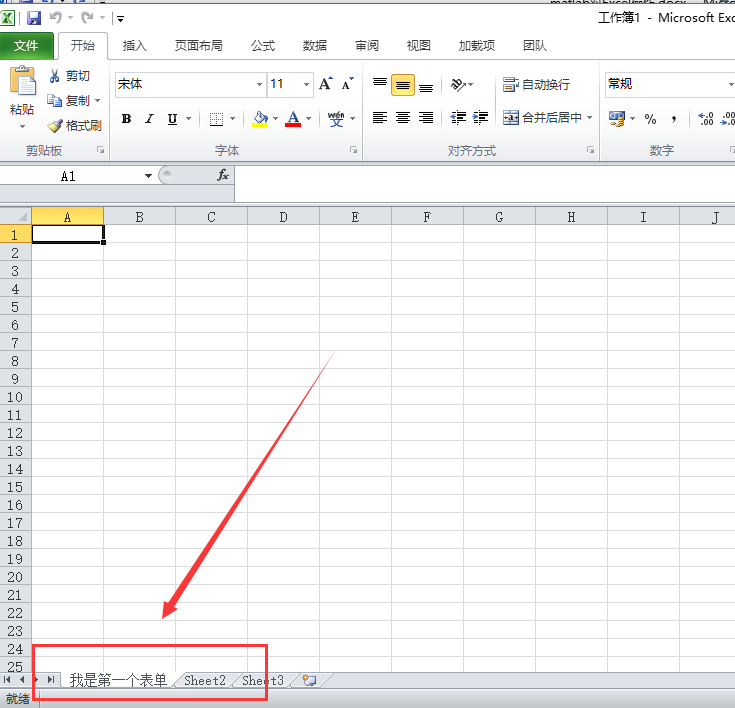图3

5.页面设置

Sheet1.PageSetup.TopMargin = 60;       % 上边距40磅
Sheet1.PageSetup.BottomMargin = 45;    % 下边距40磅
Sheet1.PageSetup.LeftMargin = 45;      % 左边距40磅
Sheet1.PageSetup.RightMargin = 45;     % 右边距40磅

PageSetup属性：

1. TopMargin        上边距
2. BottomMargin   下边距
3. LeftMargin         左边距
4. RightMargin      右边距

6. 设置行高列宽

RowHeight = [25,25,25,25]';
Sheet1.Range('A1:A4').RowHeight = RowHeight;
Sheet1.Range('A1:H1').ColumnWidth = [10,8,8,8,8,8,8,8,20];

属性设置

1. RowHeight属性表示：行高
2. ColumnWidth属性表示:列宽
3. Range:设置表格范围。详解如下

Range('A1:A16')表示：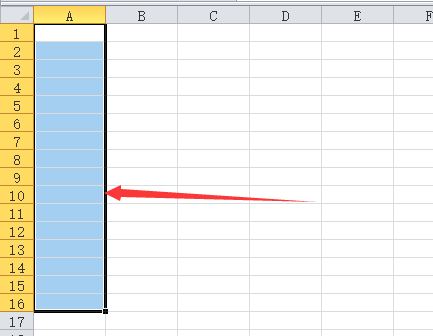Range('A1:B6')表示：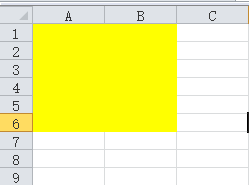7.单元格子合并

MergeCells属性表示：合并单元格

Sheet1.Range('A1:H1').MergeCells = 1;
Sheet1.Range('A2:H2').MergeCells = 1;
Sheet1.Range('A8:A9').MergeCells = 1;
Sheet1.Range('B8:D8').MergeCells = 1;
Sheet1.Range('E8:H8').MergeCells = 1;
Sheet1.Range('B9:D9').MergeCells = 1;
Sheet1.Range('E9:H9').MergeCells = 1;
Sheet1.Range('A10:H10').MergeCells = 1;
Sheet1.Range('A11:H11').MergeCells = 1;
Sheet1.Range('A12:H12').MergeCells = 1;
Sheet1.Range('A13:H13').MergeCells = 1;
Sheet1.Range('A14:H14').MergeCells = 1;
Sheet1.Range('D16:H16').MergeCells = 1;

MergeCells详解见https://docs.microsoft.com/zh-cn/office/vba/api/excel.range.mergecells

8.设置单元格的边框

Sheet1.Range('A4:H14').Borders.Weight = 3;
Sheet1.Range('A10:H12').Borders.Item(3).Linestyle = 0;
Sheet1.Range('A10:H12').Borders.Item(4).Linestyle = 0;
Sheet1.Range('A13:H13').Borders.Item(4).Linestyle = 0;
Sheet1.Range('A14:H14').Borders.Item(3).Linestyle = 0;

Borders边框属性：

1. Weight：宽度
2. Linestyle：添加双边框

9.设置单元格对齐方式

Sheet.Range('A1:C3').HorizontalAlignment = -4108;   % 水平居中
Sheet.Range('A1:C3').VerticalAlignment = -4160;     % 顶部对齐

1. HorizontalAlignment属性表示：水平居中
2. VerticalAlignment属性表示：顶部对齐

这两个对齐方式等于的那个值是枚举值，具体可以参考微软官网

https://docs.microsoft.com/zh-cn/office/vba/api/overview/excel/enumerations-graph-visual-basic-reference

10.写入单元格内容（Value）

Sheet1.Range(范围).Value = '插入的信息';

11.设置字号样式

Sheet1.Range('A4:H12').Font.size = 10.5;    % 设置单元格A4至H12的字号为10.5
Sheet1.Range('A1').Font.size = 16;          % 设置单元格A1的字号为16
Sheet1.Range('A1').Font.bold = 2;           % 单元格A1的字体加粗
Sheet.Range('A1:C3').Font.Name = '华文行楷'

Font属性详细见：https://docs.microsoft.com/zh-CN/office/vba/api/excel.font(object)

12. 保存WorkBook和退出Excel服务端

WorkBook.SaveAs([pwd, '\我的第一个excel']);%pwd表示路径，保存“我的第一个excel”
Excel.Quit%退出

clear
clc

file_path=[pwd,'\test.xlsx']%设置当前路径
try
Excel=actxGetRunningServer('Excel.Application')%如果Excel 服务器已经打开，返回其句柄
catch
Excel=actxserver('Excel.Application')%如果Excel服务器没有打开，则创建一个Excel服务器，并返回句柄
end
Excel.Visible = 1; ;%设置Excel服务器为可见状态

%如果存在test.xlsx文件,则打开文件，若不存在则创建一个,然后保存
if exist(file_path,'file')
Workbook=Excel.Workbooks.Open(file_path)%打开
else
Workbook.SaveAs(file_path)%保存
end

% 返回当前工作表句柄
Sheets = Excel.ActiveWorkbook.Sheets;
Sheet1 = Sheets.Item(1);    % 返回第1个表格句柄
Sheet1.Activate;    % 激活第1个表格

% 页面设置
Sheet1.PageSetup.TopMargin = 60;       % 上边距40磅
Sheet1.PageSetup.BottomMargin = 45;    % 下边距40磅
Sheet1.PageSetup.LeftMargin = 45;      % 左边距40磅
Sheet1.PageSetup.RightMargin = 45;     % 右边距40磅

% 设置行高和列宽
% 定义行高向量RowHeight
RowHeight = [25,25,25,25]';
% 设置Range对象（从A1到A16）的行高
Sheet1.Range('A1:A4').RowHeight = RowHeight;
% 设置Range对象（从A1到H1）的列宽
Sheet1.Range('A1:H1').ColumnWidth = [10,8,8,8,8,8,8,8,20];

% 合并单元格
Sheet1.Range('A1:A4').MergeCells = 1;
Sheet1.Range('B1:C1').MergeCells = 1;
Sheet1.Range('B2:C2').MergeCells = 1;
Sheet1.Range('B3:C3').MergeCells = 1;
Sheet1.Range('B4:C4').MergeCells = 1;
Sheet1.Range('A8:A9').MergeCells = 1;
Sheet1.Range('D3:E3').MergeCells = 1;
Sheet1.Range('E8:H8').MergeCells = 1;
Sheet1.Range('D4:E4').MergeCells = 1;
Sheet1.Range('G4:H4').MergeCells = 1;
Sheet1.Range('I1:I4').MergeCells = 1;

% 设置单元格的边框
Sheet1.Range('A1:I4').Borders.Weight = 3;

% 写入单元格内容
Sheet1.Range('A1:A4').Value = '毕业生信息';
Sheet1.Range('B1:C1').Value = '  姓 名  ';
Sheet1.Range('A2:C2').Value = '政治面貌';
Sheet1.Range('A3:C3').Value = '通讯地址';
Sheet1.Range('A4:C4').Value = 'E-mail';
Sheet1.Range('E1').Value = '性别';
Sheet1.Range('E2').Value = '健康状况';
Sheet1.Range('F4').Value = '现居住地址';
Sheet1.Range('G1').Value = '出生年月';
Sheet1.Range('G2').Value = '联系电话';
Sheet1.Range('G3').Value = '邮政编码';展开全文matlab
• 怎么把matlab中处理的数据存入到excel中1.上双开matlab2.接着可以直接在命进行相关的操作3.也可以直接新建一个脚本，我这里是直接在命令行进行的4.现在我们介绍一下关于将数据变量导出到excel中使用的函数xlswrite的...
• matlab 建立excel表格----例子

万次阅读 2016-09-08 10:28:49
function ceshi_Excel ...%利用MATLAB生成Excel文档 % ceshi_Excel % % Copyright 2009 - 2010 xiezhh.  % $Revision: 1.0.0.0$ $Date: 2009/12/14 20:40:00$ filespec_user
• 如何将excel中数据导入matlab并存入数组中具体操作步骤如下：1、首先，打Matlab软件，在顶部菜单栏上找到“import data”，单击打开，如下图所示，然后进入下一步。2、其次，在弹出页面中，如下图所示，要导入的...
• 大学时候选修Matlab，算是有了入门基础。现在工作了偶尔需要用到，将各种数据绘图进行对比，因此又安装使用上。------------------------分界线-以下正文-------------------现在有一个Excel数据文件，是一组时域...
• excel数据读取函数：[num, txt, raw, X] = xlsread(filename, sheet, range)filename, sheet, range 文件名，sheet名、范围num, txt, raw, X 输出excel数据写入函数：[status, message] =xlswrite(filename, M, ...
• MATLAB 无法写入Excel解决方法

千次阅读 2018-08-29 23:54:08
https://blog.csdn.net/Seis_juch/article/details/71435961
• 01功能介绍excel是我们常用的一款电子表格软件，其可以直观的展现我们收集的数据，并对其进行数据...而对于像作者一样，不懂vba但会matlab或python语言的使用者可以使用另一种方，即将excel的数据读入另外的编程软件...
• matlab中读取excel数据格式类型和直接在界面输入的数据形成的格式不一样，怎么才能用excel读取成功？那个‘abc’等非字符串是导入的吧，如果你要导入的是纯数字的，以告诉你，但是你的txt文件先调一下，像 12 33 36 ...
• 3、新建MATLAB脚本 clc; %清理命令行窗口 clear all; %清理工作区 %读取Excel文件 tx = xlsread('C:\Users\Lihongda‘s PC\Desktop\data.xls'); %电机目标速度 Mts = tx(:,1); %电机实际速度 Ams = tx(:,2); plot...matlab excel
• Matlab读取Excel各个子表的数据进行拟合 前言 现有一个Excel文件，里面存有很多个子表，每个子表格式一样，但是数据不一样，现要对每个子表中的好多行数据进行多项式拟合，并将拟合好的结果保存回Excel中各个子...matlab 数据拟合
• 如何用matlab导入excel数据并读取1、很简单的用xlswrite函数就了先打开matlab，输入你的代码2、找到你要存件的位置复制绝径(致谢文件名的话就会存放在当前目录中)，如图以f盘根目录为例)3、然后写xlswrite函数，格式...
• Excel文档写入数据：xlswrite函数：格式为：...A表示MATLAB工作空间中的数据，将A保存到Excel表中；sheet表示数据A保存在文件的哪一张表中，如果sheet为已存在的表名称，则存入，若Excel文件中没有该名称的...
• 怎样将matlab中的数据导入到excel中1、首先打matlab新建窗口。2、在matlab中生成数据，并且写入到excel文件中体代码如下图所示。3、下面一下生成的myExample.xlsx文件里面是否含有matlab所生成的数据。4、图中可以...
• 如何将Excel数据导入MATLAB中“打开”弹出“import”窗口，在窗口工具侧导入选项中选择“matrix(矩阵)”，然后点击工具栏右侧的“勾”，导入数据关闭“import”窗口，回到matlab主程序，所以如果经常用到该变量，在...
• 其中，progid为该组件的进程句柄，例如，创建一个matlab实例对象，progid为：matlab.application创建一个word对象，progid为：word.application创建一个excel对象，progid为:excel.application总之，创建一...matlab定义对象matlab 开发语言
• 你这个似乎用不着用matlab来求，用matlab来求反倒是增加了一个环节。直接用数据透视表只需简单点几下鼠标就可得到结果。不过你的日期这样输入很不规范，无法直接使用Excel的日期数据来处理你的数据。当然对本问题的...
• 13Matlabexcel、txt的交互，包括读取，保存，新建matlab
• 如何利用matlab根据excel表格里面的数据画图将待导入的矩阵结数据Excel中，录入时注意行列原矩阵一一对应录入完以后数据，为了后续步骤使用方便，命名时我们最好把它命名为我们接下来在MATLAB中要引用的变量名(比如...matlab引用数据
• 首先，我的声明一下，我不是专门学习matlab的，我是学隧道工程的，所以我不专业，我主要学习的是matlab中一些智能算法的y回归预测，其他的没有学习，我只是想把自己所学的东西写一写，以便今后自己的学习，所以大家...
• 本章内容为用matlab打开Excel，添加 (打开) 工作簿，添加工作表，更改工作表名称，向Excel写入数据，保存文件等。matlab Excel...

matlab新建excelmatlab 订阅